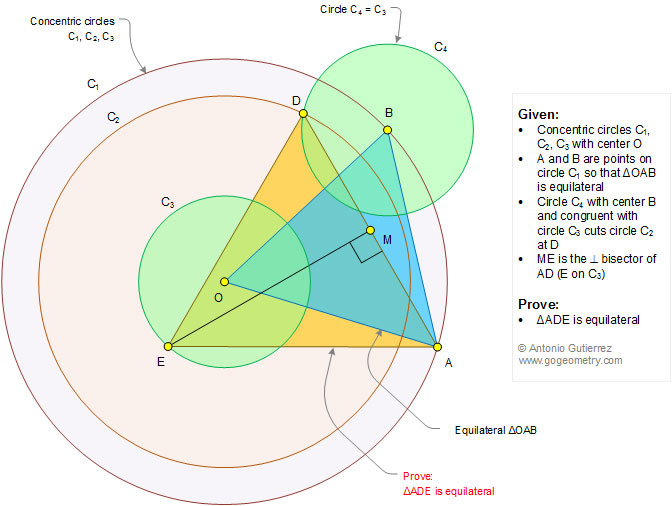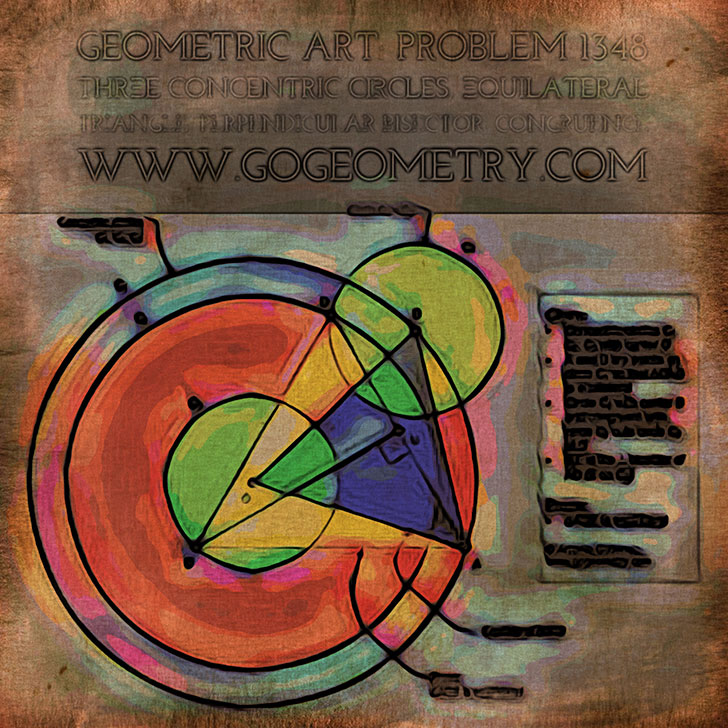# Geometry Problem 1348: Three Concentric Circles, Equilateral Triangle, Perpendicular Bisector, Congruence. Math Infographic.

### Proposition

The figure shows three concentric circles C1, C2, and C3 with center O. Points A and B are on circle C1 so that the triangle OAB is equilateral. Circle C4 with center B and congruent with circle C3 cuts circle C2 at D. ME is the perpendicular bisector of AD (E on C3). Prove that the triangle ADE is equilateral.## Geometric Art of Problem 1348 using iPad Apps